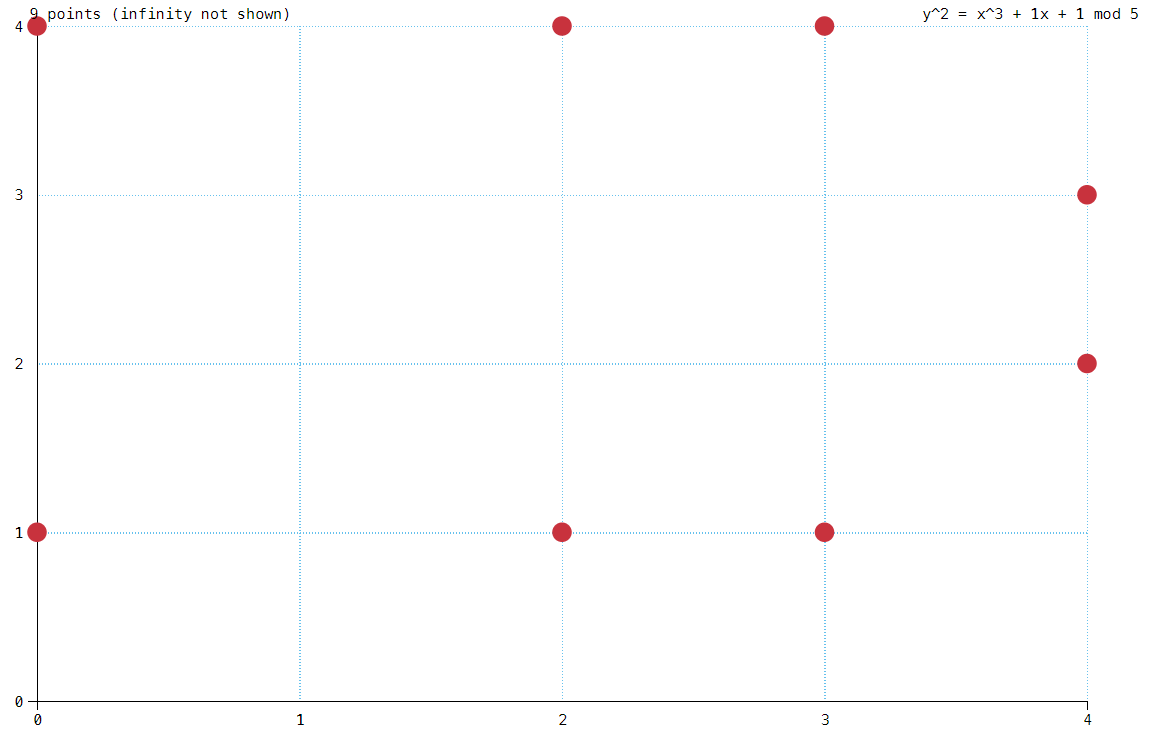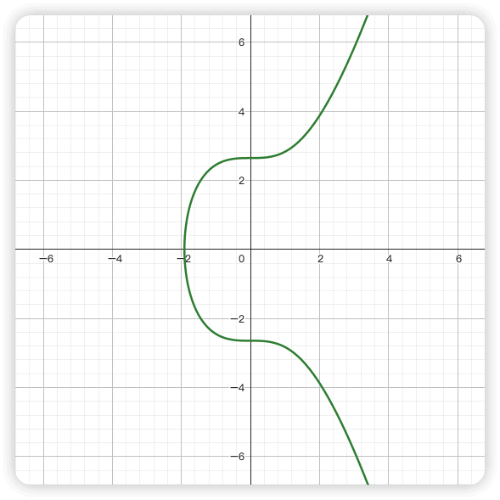# 椭圆曲线加密算法

· 阅读需 15 分钟

## 椭圆曲线定义​

$y^2 = x^3 + ax +b，4a^3 + 27b^2 \neq 0$## 椭圆曲线的四则运算​

### 加法​$P=Q$时，相当于过点 P 做曲线的切线，相交于点$R$$R$ 关于 X 轴的对称点 $R'$ 就是相加得到的点。 $P + P = 2P = R'$$Q=-P$时，即$Q$点是$P$点在椭圆曲线上关于 X 轴的对称点，此时过这两点做直线。没有相交的第三个点。假设$P+Q = O$，则$P + Q = P + (-P) = O$$O$是一个无穷远点，也叫加法的单位元。曲线上任意点与单位元相加都等于其自身。 $P + O = P$### 减法​

$P-Q$ 可以看成$P + (-Q)$### 乘法​## 有限域椭圆曲线​

• 加法：$a + b = c \ mod \ p$
• 减法：$a-b = a + (-b) \ mod\ p$
• 乘法：$a * b = c \ mod \ p$
• 除法：$a \div b = a \times b ^ {-1}=c \ mod\ p$

• $y^2 = x^3 + ax + b$ 上的所有点的 $x$$y$ 坐标值是$𝔽_p$中的两个整数，因此有限域椭圆曲线图是一定数量的点形成的图
• $a$$b$ 是满足$4a^3 + 27b^2 \neq 0$且在$𝔽_p$中的两个整数

• $x=0, y=1$, 左右两边对 5 取余结果都为 1，该点有效
• $x = 0, y = 2$, 左边对 5 取余结果为 4，右边对 5 取余结果为 1，两边不等，该点无效
• $x = 0, y = 4$, 左右两边对 5 取余结果都为 1，该点有效### 有限域椭圆曲线加法​$P=(16, 20)$, 点$Q =(41,120)$$P+Q$ 的坐标点$R'(x'， y')$

$x_R = k^2 - x_P - x_Q \ mod\ p = 16-16-41 \ mod\ 127 = -41 \ mod\ 127 = 86$

$y_R=[y_P + k(x_R-x_P)] \ mod\ p = [20 + 4(86 - 16)]\ mod\ 127 = 300\ mod\ 127 = 46$

$R'$$R$关于$x$轴的对称点，所以

$x_{R'} = x_R = 86， y_{R'}=-y_R \ mod\ p = -46 \mod \ 127 = 81$

### 有限域椭圆曲线乘法​

• $0P = 0$
• $1P = (3, 6)$
• $2P = P + P = (80, 10)$
• $3P=2P+P=(80, 87)$
• $4P=3P+P(3, 91)$
• $5P = 4P+P=0$
• $6P=5P+P=(3, 6)$
• $7P =6P+P =(80, 10)$
• $\cdots$$P$的阶和椭圆曲线是有联系的，拉格朗日定理告诉我们，子群的阶是父群的阶的因子。换句话说，如果一个椭圆曲线包含 $N$ 个点，它的一个子群包含 $n$ 个点，那么 $n$$N$ 的因子

• 计算椭圆曲线的阶$N$ （上面定义了阶是点集的数量，因此$N$ 就是有限域上椭圆曲线的有效点的数量）
• 找出$N$的所有因子
• 对每个因子计算$nP$
• 找到最小的且满足 $nP=0$$n$, $n$ 就是子群的阶$1P\neq 0, 2P\neq0,\cdots,7P=0$, 因此$P$的阶是 7。

## 椭圆曲线加密算法​

### ECDH​

ECDH 是椭圆曲线的笛福赫尔曼算法的变种，它其实不单单是一种加密算法，而是一种密钥协商协议， 也就是说 ECDH 定义了密钥怎么样在通信双方之间生成和交换。

• A 选择了一条有限域上椭圆曲线$E_p(a,b)$ 并选择了一个基点$G$, 生成了自己的公钥和私钥。之后将自己的椭圆曲线$E_p(a,b)$、基点$G$ 和公钥传给 B。B 根据这些信息生成自己的公钥和私钥
• A 的私钥记作$k_A$，公钥$H_A = k_AG$
• B 的私钥记作$k_B$，公钥$H_B = k_BG$
• A 和 B 交换各自的公钥
• A 计算共享秘钥$S=k_AH_B$（使用自身的私钥和 B 的公钥）, B 计算共享秘钥$S=k_BH_A$（使用自身的私钥和 A 的公钥）, A 和 B 计算得到的$S$ 是相同的，因为$S=k_AH_B = k_A(k_BG) = k_B(k_AG)=k_BH_A$
• 使用共享秘钥来加密数据进行通信。

### ECDSA​

A 首先生成自己的公钥和私钥，即从$\{1,\cdots,n-1\}$随机选择一个数$k_A$作为私钥，公钥 $H_A=k_AG$

A 生成签名过程如下

• $\{1,\cdots,n-1\}$ 中选择一个随机数$k$
• 计算 $P(x, y)=kG$
• 计算$r = x_P\ mod\ n$$x_P$$P$点的$x$坐标），若$r=0$, 则重新选择一个$k$ 并重新计算
• 计算$s=k^{-1} (h+rk_A) \ mod\ n$$k_A$是 A 的私钥，$k^{-1}$$k$的乘法逆元， h 是要发送消息的哈希），若$s=0$ ,则重新选择一个$k$并重新计算

B 验证签名过程如下:

• 计算$u_1 = s^{-1}h \ mod \ n$
• 计算$u_2 = s^{-1}r \ mod \ n$
• 计算$P = u_1G + u_2H_A$, 当 $r = x_P \ mod \ n$$x_P为$$P$$x$坐标），则验证成功

$P = u_1G + u_2H_A = u_1G + u_2k_AG = (u_1 + u_2k_A)G = (s^{-1}h + s^{-1}rk_A)G = (h+rk_A)s^{-1}G$

ECDSA 实践

• 椭圆曲线$E_{29}(4,20): y^2=x^3+4x+20 \ mod\ 29$
• 基点$G=(2, 6)$$G$的阶$n=37$
• 消息$h=Hash(message) = 88$

A 签名

• $[1,36]$随机选择一个整数 7 作为私钥，则公钥$H=kG=7(2,6)=(3,28)$
• $[1, 36]$随机生成一个整数$k=11$
• 计算$P=kG = 11(2, 6) = (16,2)$
• 计算$r=x_P\ mod\ n = 16 \ mod\ 37 = 16$
• 计算$s = k^{-1} (h+rk_A) \ mod\ n = k^{-1}(88+16*7) \ mod\ n=200k^{-1} \ mod \ 37 = 200 * 27 \ mod\ 37 = 35$
• 发送$(r, s)$ 、A 的公钥$H_A$、消息$h$ 给 B

B 验证签名

• 计算$u_1=s^{-1}h \ mod \ n = 18 * 88 \ mod\ 37=30$
• 计算$u_2 = s^{-1}r \ mod \ n = 18 * 16\ mod\ 37 = 29$
• 计算$R = u_1G + u_2H_A = 30(2, 8) + 29(3, 28) = (16, 2)$
• 计算 $x_R \ mod\ n = 16 \ mod\ 37 = 16 = r$, 说明签名合法

### secp256k1​

secp256k1 是一条特定的椭圆曲线，椭圆曲线方程中 a =0, b = 7，故方程为$y^2 = x^3 + 7$。曲线图如下：$p=2^{256} - 2^{32}-2^{9}-2^8-2^7-2^6-2^4-1$

= 0xfffffffffffffffffffffffffffffffffffffffffffffffffffffffefffffc2f

$G$的阶

$n = 2^{256}-4324203865659656852420866394968145599$

=0xfffffffffffffffffffffffffffffffebaaedce6af48a03bbfd25e8cd0364141

[1, n-1] 随机取一个数$k$作为私钥，则公钥$H=kG$

• ecc: 如何生成公私钥
• ecdh：通信双方如何生成相同的加密秘钥
• ecdsa：对通信的消息如何签名才能确保消息没有被篡改
• secp256k1：特定的椭圆曲线